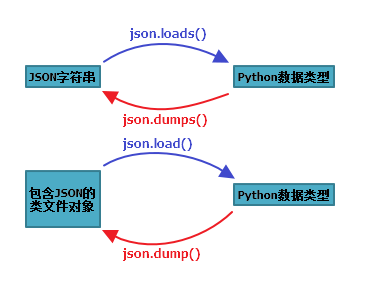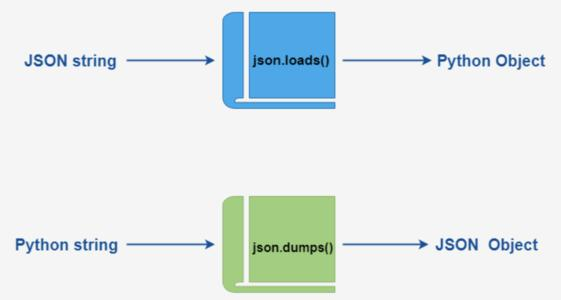• ## 使用Python解析JSON

万次阅读 多人点赞 2019-04-13 12:44:02
Python3 中可以使用 json 模块来对 JSON 数据进行编解码，主要包含了下面4个操作函数： 提示：所谓类文件对象指那些具有read()或者 write()方法的对象，例如，f = open('a.txt','r')，其中的f有read()方法，所以f...
JSON (JavaScript Object Notation) 是一种轻量级的数据交换格式。Python3 中可以使用 json 模块来对 JSON 数据进行编解码，主要包含了下面4个操作函数：提示：所谓类文件对象指那些具有read()或者 write()方法的对象，例如，f = open('a.txt','r')，其中的f有read()方法，所以f就是类文件对象。

在json的编解码过程中，python 的原始类型与JSON类型会相互转换，具体的转化对照如下：

Python 编码为 JSON 类型转换对应表：

Python
JSON
dict
object
list, tuple
array
str
string
int, float, int- & float-derived Enums
number
True
true
False
false
None
null
JSON 解码为 Python 类型转换对应表：

JSON
Python
object
dict
array
list
string
str
number (int)
int
number (real)
float
true
True
false
False
null
None
操作示例 ：

import json

data = {
'name': 'pengjunlee',
'age': 32,
'vip': True,
'address': {'province': 'GuangDong', 'city': 'ShenZhen'}
}
# 将 Python 字典类型转换为 JSON 对象
json_str = json.dumps(data)
print(json_str) # 结果 {"name": "pengjunlee", "age": 32, "vip": true, "address": {"province": "GuangDong", "city": "ShenZhen"}}

# 将 JSON 对象类型转换为 Python 字典
user_dic = json.loads(json_str)
print(user_dic['address']) # 结果 {'province': 'GuangDong', 'city': 'ShenZhen'}

# 将 Python 字典直接输出到文件
with open('pengjunlee.json', 'w', encoding='utf-8') as f:
json.dump(user_dic, f, ensure_ascii=False, indent=4)

# 将类文件对象中的JSON字符串直接转换成 Python 字典
with open('pengjunlee.json', 'r', encoding='utf-8') as f:
ret_dic = json.load(f)
print(type(ret_dic)) # 结果 <class 'dict'>
print(ret_dic['name']) # 结果 pengjunlee

注意：使用eval()能够实现简单的字符串和Python类型的转化。

user1 = eval('{"name":"pengjunlee"}')
print(user1['name']) # 结果 pengjunlee


展开全文json
• ## Python解析JSON对象

千次阅读 多人点赞 2021-03-23 13:25:32
Python解析JSON对象 本章节我们将为大家介绍如何使用 Python 语言来编码和解码 JSON 对象。 json处理模块的主要任务，是将一个JSON对象，转换成Python数据类型数据进行处理，或者反之，将Python数据类型数据，...
Python解析JSON对象

本章节我们将为大家介绍如何使用 Python 语言来编码和解码 JSON 对象。json处理模块的主要任务，是将一个JSON对象，转换成Python数据类型数据进行处理，或者反之，将Python数据类型数据，转换成JSON对象（字符串流），在不同的模块或者系统间传输。

文章目录Python解析JSON对象1. JSON数据格式特点2. 常用方法总结3. 序列化和反序列化3.1 序列化3.2 反序列化
1. JSON数据格式特点

对象表示为键值对
数据由逗号分隔
花括号保存对象
方括号保存数组

{
"students": [
{ "name":"北山啦" , "age":20 },
{ "name":"张三" , "age":30 },
{ "name":"里斯" , "age":17 }
]
}

{'students': [{'name': '北山啦', 'age': 20},
{'name': '张三', 'age': 30},
{'name': '里斯', 'age': 17}]}

上面就是一个JSON格式数据。它开起来就像是在Python中的字典数据类型。我们可以通过json模块将它转换成字符串或者反过来将字符串转换成字典数据类型。
JSON也支持各种数据类型，它的数据类型和Python各种数据类型之间的对比如下：

object —— dict
array —— list
string —— str
number —— int/float
true/false —— True/False
null —— None

2. 常用方法总结
在json模块中，用于处理json的主要是四个函数，分别是：

loads()：从JSON字符串中读取数据并转换成Python数据类型
load()：从JSON文件中读取数据并转换成Python数据类型
dumps()：将Python数据类型数据转换成JSON字符串
dump():将Python数据类型数据转换成JSON字符串写入到文件

3. 序列化和反序列化从JSON数据转换到Python数据，叫反系列化（deserialization）
从Python数据转换到JSON数据，叫系列化（serialization）

3.1 序列化

系列化:将Python数据转换成JSON字符串的方法。

下面我们先来看一个简单的例子。
import json

data = {
"students": [
{ "name":"北山啦" , "age":20 },
{ "name":"张三" , "age":30 },
{ "name":"里斯" , "age":17 }
]
}
print(type(data))
print(data)
json_str = json.dumps(data)
print(type(json_str))

<class 'dict'>
{'students': [{'name': '北山啦', 'age': 20}, {'name': '张三', 'age': 30}, {'name': '里斯', 'age': 17}]}
<class 'str'>

上面的例子中，虽然看起来数据没有发生变化，但其实它们的数据类型已经发生了本质的改变：将字典数据类型的data，转换成了str类型，然后我们就可以将这个str类型的数据转换成流，在网络上进行传输或者写入到文件等。
import json

data = {
"students": [
{ "name":"北山啦" , "age":20 },
{ "name":"张三" , "age":30 },
{ "name":"里斯" , "age":17 }
]
}

print(type(data))
print(data)
json_str = json.dumps(data, separators=('>>','::'), indent=2)
print(json_str)

<class 'dict'>
{'students': [{'name': '北山啦', 'age': 20}, {'name': '张三', 'age': 30}, {'name': '里斯', 'age': 17}]}
{
"students"::[
{
"name"::"\u5317\u5c71\u5566">>
"age"::20
}>>
{
"name"::"\u5f20\u4e09">>
"age"::30
}>>
{
"name"::"\u91cc\u65af">>
"age"::17
}
]
}

将data写入txt文件中
import json

data = {
"students": [
{ "name":"北山啦" , "age":20 },
{ "name":"张三" , "age":30 },
{ "name":"里斯" , "age":17 }
]
}
with open("students.txt","w") as fp:
json.dump(data, fp, ensure_ascii=False)
print("finish")

finish

这样就将data写入了students.txt，看看是不是已经将数据写进去了。
3.2 反序列化

从JSON数据转换到Python类型数据，叫反系列化。可以通过loads()/load()这两个方法来完成。

import json
with open("students.txt") as fp:
data = json.load(fp)
"""取出字典key为students的数据，
得到一个list，再从这个list中取第一个数据"""
print(data['students'])

{'name': '北山啦', 'age': 20}


parse_int参数

默认值为None,如果指定了parse_int，用来对JSON int字符串进行解码,这可以用于为JSON整数使用另一种数据类型或解析器。

parse_int参数，这里我们简单将其指定为float类型。
import json
with open("students.txt") as fp:
data = json.load(fp, parse_int = float)
print(data)

{'students': [{'name': '北山啦', 'age': 20.0}, {'name': '张三', 'age': 30.0}, {'name': '里斯', 'age': 17.0}]}

可以看到，age原来是整数类型，通过parse_int已经被转换成了float类型。

object_hook

默认值为None,object_hook是一个可选函数，此功能可用于实现自定义解码器。指定一个函数，该函数负责把反序列化后的基本类型对象转换成自定义类型的对象。

def fromJSON(dct):  # 这里会对所有的字典数据类型都进行遍历
if isinstance(dct, dict) and 'students' in dct:
return dct['students']
else:
return Student(dct['name'], dct['age'])

import json

with open("students.txt") as fp:
data = json.load(fp, object_hook=fromJSON)
print(data)


[姓名: 北山啦, 年龄: 20, 姓名: 张三, 年龄: 30, 姓名: 里斯, 年龄: 17]到这里就结束了，如果对你有帮助，欢迎点赞关注评论，你的点赞对我很重要


展开全文json
• ## python解析excel函数

万次阅读 2019-08-15 00:18:22
python解析excel函数 在现在的开发的工作中，随着数据的重要性日益凸显，经常需要与excel文件打交道，目前的大多数第三方库只提供读取excel文件的方法，但有时候需要将预先定义好的 “excel函数” 插入到excel文件...
python解析excel函数

目录

python解析excel函数

目前主流解析excel文件的第三方库：

本文主要使用的第三方库：

具体代码：

Notes：

在现在的开发的工作中，随着数据的重要性日益凸显，经常需要与excel文件打交道，目前的大多数第三方库只提供读取excel文件的方法，但有时候需要将预先定义好的 “excel函数” 插入到excel文件中，此时大多数第三方库无法支持。

本文中，主要提供一种解决这类问题的思路，并简单举例

目前主流解析excel文件的第三方库：

xlrd、xlwt、xlutils：只能处理xls格式，不能处理xlsx格式，不能读取公式，只能读取公式计算后的数值，这样保存后的excel文件丢失了公式。
openpyxl：以处理xlsx格式，使用data_only=False参数打开xlsx格式，能读取公式；data_only=True读取公式的数值，注意：openpyxl 本身无法解析 excel公式并进行运算，但可以通过更改原本excel公式中的变量数值（单元格的数值）实现计算的功能。（excel软件保存文件时，会记录公式、数值和运算）
xlsxwriter：理可以写公式，但是不能读取excel文件，只能用于创建新的excel文件。

本文主要使用的第三方库：

formulas：实现的excel函数的解析功能，并且可以高度自定义自己的公式，本文中，主要通过重写excel基础函数的功能实现解析excel函数的功能。（链接地址：https://formulas.readthedocs.io/en/stable/doc.html）

具体代码：

# -*- coding: utf-8 -*-
import math
import formulas

class ParseFormulas:
"""
此示例主要通过自定义公式的方法讲述如何解析excel公式，具体在excel中使用可以参考formulas标准库的使用
"""

def __init__(self, formulas_str):
self._formulas_str = formulas_str
self._base_formulas()

def _base_formulas(self):
"""
定义excel的基础函数逻辑
:return:
"""
base_func = formulas.get_functions()
base_func['ROUNDUP'] = lambda x: math.ceil(x)
base_func['MAX'] = lambda *x: max(list(x))
base_func['MIN'] = lambda *x: min(list(x))
base_func['IF'] = lambda x, t, f: self._base_formulas_if(x, t_value=t, f_value=f)
base_func['AND'] = lambda x, y, *z: self._base_formulas_and((x, y), *z)
base_func['OR'] = lambda x, y, *z: self._base_formulas_or((x, y), *z)

@staticmethod
def _base_formulas_and(judge_tuple, *args):
"""
Excel AND 函数的解析逻辑
:param judge_tuple: 判断条件（最少两个）
:param args: 两个之外的判断条件
:return: bool
"""
judge_list = [a for a in judge_tuple if not a]
judge_list.extend([b for b in args if not b])
if len(judge_list) == 0:
return True
else:
return False

@staticmethod
def _base_formulas_or(judge_tuple, *args):
"""
Excel OR 函数的解析逻辑
:param judge_tuple: 判断条件（最少两个）
:param args: 两个之外的判断条件
:return: bool
"""
judge_list = [a for a in judge_tuple if a]
judge_list.extend([b for b in args if b])
if len(judge_list) > 0:
return True
else:
return False

@staticmethod
def _base_formulas_if(judge_tuple, t_value, f_value):
"""
Excel IF 函数的解析逻辑
:param judge_tuple: 判断条件
:param t_value: 条件成立的返回值
:param f_value: 条件不成立的返回值
:return:
"""
return t_value if judge_tuple else f_value

def new_formulas(self):
"""
解析输入的公式
:return: 公式计算结果
"""
new_func = formulas.Parser().ast(self._formulas_str).compile()
print('formulas_value: ', new_func())
return new_func()

if __name__ == '__main__':
formulas_str0 = '=AND(2>1, 3<4)'
formulas_str1 = '=MAX(1,4,8,5,MIN(12, 15), ROUNDUP(12.346))'
formulas_str2 = '=MIN(2,6,12,7)'
formulas_str3 = '=OR(2>1, 5<7, 12>6,)'
formulas_str4 = '=ROUNDUP(1.145)'
formulas_str5 = '=MIN(2,6,12,7)'
formulas_str6 = '=IF(OR(AND(1<0, 2>3), OR(4>7, 6>7)),MAX(ROUNDUP(12.32),2, 9),7)'

ParseFormulas(formulas_str0).new_formulas()
ParseFormulas(formulas_str1).new_formulas()
ParseFormulas(formulas_str2).new_formulas()
ParseFormulas(formulas_str3).new_formulas()
ParseFormulas(formulas_str4).new_formulas()
ParseFormulas(formulas_str5).new_formulas()
ParseFormulas(formulas_str6).new_formulas()

"""
运行结果：
formulas_value:  True
formulas_value:  13
formulas_value:  2
formulas_value:  True
formulas_value:  2
formulas_value:  2
formulas_value:  7
"""

Notes：

openpyxl 开启 data_only=False 时，如果是通过 openpyxl 创建的且有输入excel公式（字符串），通过excel软件是可以看到 公式的计算数值的，此时不要以为openpyxl 可以解析excel公式，实际上是excel软件解析了 excel公式 并计算出结果。如果不通过excel软件再次保存，再次通过data_only=True 查看数值时，只会得到None，而不是计算后的数据，openpyxl本身无法解析excel函数。
示例：
# -*- coding: utf-8 -*-
import openpyxl

class ExcelOpener:

def __init__(self, f_path):
self.f_path = f_path
self.creater()
self.opener()

def creater(self):
"""
创建 excel 文件
:return:
"""
wb = openpyxl.Workbook()
sheet = wb.active
sheet["A1"] = 200
sheet["A2"] = 300
sheet["A3"] = 200
sheet["A4"] = 500
sheet["A5"] = "=SUM(A1:A4)"
wb.save(self.f_path)

def opener(self):
"""
读取 excel 文件
:return:
"""
# 展示公式， data_only 默认为 False
wbFormulas = openpyxl.load_workbook(self.f_path)
sheet = wbFormulas.active
print(sheet["A5"].value)
# 结果： =SUM(A1:A4)

# 展示数值
wbDataOnly = openpyxl.load_workbook(self.f_path, data_only=True)
sheet = wbDataOnly.active
x = (sheet["A5"].value)
print(x)
# 结果：None? 而不是 1200?

if __name__ == '__main__':
f_path = "writeFormula.xlsx"
ExcelOpener(f_path)



展开全文• ## python解析域名

千次阅读 2017-12-14 17:58:22
python解析域名
解析域名主要用到gethostbyname 函数
具体示例如下：
def parsing_domain_name():
##parsing domain names
import socket

try:
hostip =socket.gethostbyname('www.baidu.com')#解析百度的域名所对应的ip，gethostbyname主要解析单个域名
print(hostip)
except socket.error as e:
print("gethostbyname failed")
return hostip                                    #函数返回值为解析完的ip

print(parsing_domain_name())#打印解析完毕的ip

解析多个域名可以选择socket.getnameinfo()函数，流程如上

展开全文socket 函数
• 本课程是面向"超级小白"的课程。本课程希望通过一种兴趣的形式... 本课程主要有的内容： 讲解多个编程语言的适用的领域，以及各自的特点 讲解如何安装python解析器 讲解通过模块turtle如何绘制各种图形 后续的扩展学习
• 如果某些特殊原因（比如有些框架只能在Python2.7中使用），需要修改程序在 Python2.7 下运行，即可设置Python解析器为 Python2.7。 在菜单栏中依次选择 File → Setting → Project : go big(这里是项目名称) → ...
• ## Python解析器

千次阅读 2013-11-06 12:23:45
嵌入Python解析器执行一些简单的Python 脚本脚本很容易，但是当python解析器用到扩展模块时和多个线程都需要Python解析器执行脚本时，遇到了一些麻烦问题，下面是在查看帮助文档过程中，一些简单的翻译记录： ...
• 这篇博客内容将包括对XML文件的解析、追加新元素后写入到XML，以及更新原XML文件中某结点的值。使用的是python的xml.dom.minidom包，详情可见其官方文档：xml.dom.minidom官方文档。全文都将围绕以下的customer.xml...xml
• ## 如何用python解析mnist图片

万次阅读 多人点赞 2015-08-12 15:48:18数据结构 图片
• 文章目录一、json介绍二、用python解析json文件 一、json介绍 JSON(JavaScript Object Notation)是一种轻量级的数据交换格式。它使得人们很容易的进行阅读和编写，同时也方便了机器进行解析和生成。它是基于...
• bpython是轻量级的Python解析器，同时包含了常见的IDE功能。功能包括语法高亮，预计参数列表、自动缩进和自动补全（下面是用法演示）。 bpython并不是一个完整的IDE，它的主要目的是为了快速把灵感用实用又轻量...
• ## python解析xml字符串

千次阅读 2017-08-28 15:13:35
在平时，经常会用到python解析xml文件，最近遇到python解析xml字符串，为大家分享 假设 data = ' 8888' 引入try: import xml.etree.cElementTree as ET except ImportError: import xml.etree.ElementTree as ET...xml
• 问题描述：由于先前安装过 python3.7.5 后面卸载了，导致 vscode 一直显示前面版本的 python3.7.5 路径，点击左下角的 select python interpreter 也找不到python 解析器。 windows python 最好选择 exe 文件安装...vscode 找不到
• 使用python解析二进制文件时，需要了解python与C语言对应的格式，如下： 　例如使用C语言保存的二进制格式，结构体为： struct Points { int x; int y; int z; }; struct Obj_info{ int id; int x1; int ...
• Python识别验证码一级目录二级目录三级目录 之前写过一篇python制作二维码的博文，受到了很多读者朋友的喜爱，也有很多读者催促我写一篇用python解析,准备了良久，写出了这篇文章。 一级目录 二级目录 三级目录 ...Python二维码 python条形码
• Python 解析pymysql模块操作数据库 pymysql 是 python 用来操作MySQL的第三方库，下面具体介绍和使用该库的基本方法。 1.建立数据库连接 通过 connect 函数中 parameter 参数 建立连接，连接成功返回Connection对象 ...数据库
• ## Python解析Las(点云)格式文件

万次阅读 多人点赞 2019-10-11 17:54:41
Python解析Las(点云)格式文件，本文代码基于python3编写。点云：在逆向工程中通过测量仪器得到的产品外观表面的点数据集合也称之为点云，通常使用三维坐标测量机所得到的点数量比较少，点与点的间距也比较大，叫稀疏...
• ## python解析json文件

万次阅读 2015-12-06 15:39:59
最近项目数据用的是json格式，学习了一下python解析json格式的方式，总结一下备忘。 文章参考链接： http://liuzhijun.iteye.com/blog/1859857 http://www.cnblogs.com/coser/archive/2011/12/14/2287739.html ...json 数据
• ## Python解析 URL

千次阅读 2019-03-30 09:57:20
Python3 中，解析URL常用的方法是 urllib.parse 模块中的 urlparse、 parse_qs 和 parse_qsl 三个函数。urlparse 将 url 分割为 scheme、netloc、path、params、query 和 fragment 这几部分，如下图所示： 在 ...URL
• Python解析json到sql语句请求一次聚合的“影讯API”就返回一串json。 使用java请求聚合的api，返回的json串们。 聚合java请求代码示例 生成的SQL 插入数据库，最终目标 decode_json.py#!/usr/bin/...json
• 这篇文章主要学习了python解析并读取PDF文件内容的方法，包括对学习库的应用，python2.7和python3.6中python解析PDF文件内容库的更新，包括对pdfminer库的详细解释和应用。主要参考了一些已有的博客内容，代码。 　...程序员 编程语言
• 转自：http://blog.chinaunix.net/uid-9525959-id-3074355.html 更多的信息，可以参考python内部的json文档： python>>> help(json) 或者官方文档： ... ...下面给出一个使用python解析json的简单例子：
• Python的JavaScript的解释器/编译器。
• python解析pdf信息，目前还是不能做到很完善，每个方法都会有它的弊端，都需要结合自己的业务场景来和其它方法一块进行使用，从而达到一个相对满意的目标。接下来主要介绍四种目前我了解到的方法（也是某博主写的，...
• ## Python解析mat文件

千次阅读 2020-04-10 16:26:08
mat文件 mat文件是matlab的数据存储的标准格式。mat文件是标准的二进制文件,还可以ASCII...很多数据集的标签都是通过mat文件来存储的，为了解析出图片所对应的的标签信息，我们需要从mat文件中来解析出这些信息， ...mat文件
• python解析xml，由于某些协会规定的原因，只能解析utf8和utf16的格式，而gbk和gb2312等解析的时候都会报错，但是对于某些xml utf-8...

# python解析python 订阅